1. A mass at the end of a string is swung in a horizontal circle at increasing speed until the string breaks.The subsequent path taken by the mass is a

2. An object rotates in a horizontal circle when acted on by a centripetal force F. What is the centripetal force acting on the object when the radius of the circle doubles and the kinetic energy of the object halves?
3. An object at the end of a wooden rod rotates in a vertical circle at a constant angular velocity. What is correct about the tension in the rod?
4. A mass is suspended by a string from a fixed point. The mass moves with constant speed along a circular path in a horizontal plane.The resultant force acting on the mass is

5. What is the acceleration of an object rotating with constant speed v in a circle of radius r?
6. A spherical planet of uniform density has three times the mass of the Earth and twice the average radius. The magnitude of the gravitational field strength at the surface of the Earth is g. What is the gravitational field strength at the surface of the planet?
7. The maximum speed with which a car can take a circular turn of radius R is v. The maximum speed with which the same car, under the same conditions, can take a circular turn of radius 2R is
8. Which single condition enables Newton’s universal law of gravitation to be used to predict the force between the Earth and the Sun?
9. On Mars, the gravitational field strength is about

1/4 of that on Earth. The mass of Earth is approximately ten times that of Mars.

What is10. A car moves at constant speed around a horizontal circular track. The resultant force on the car is always equal to
11. The weight of an object of mass 1 kg at the surface of Mars is about 4 N. The radius of Mars is about half the radius of Earth. Which of the following is the best estimate of the ratio below?12. The centres of two planets are separated by a distance R. The gravitational force between the two planets is F. What will be the force between the planets when their separation increases to 3R?
13. A mass at point X gives rise to a gravitational field strength g at point P as shown below.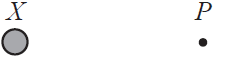An identical mass is placed at point Y as shown below.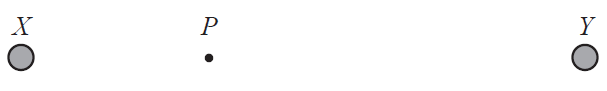The resultant gravitational field strength at P is now

14. A small sphere X of mass M is placed a distance d from a point mass. The gravitational force on sphere X is 90 N. Sphere X is removed and a second sphere Y of mass 4M is placed a distance 3d from the same point mass. The gravitational force on sphere Y is
15. The gravitational field strength at the surface of Earth is g. Another planet has double the radius of Earth and the same density as Earth. What is the gravitational field strength at the surface of this planet?
16. Newton’s law of gravitation
17. For a particle moving at constant speed in a horizontal circle, the work done by the centripetal force is
18. An object of mass m at the end of a string of length r moves in a vertical circle at a constant angular speed ω.

What is the tension in the string when the object is at the bottom of the circle?

19. A spacecraft travels away from Earth in a straight line with its motors shut down. At one instant the speed of the spacecraft is

5.4 km s–1. After a time of 600 s, the speed is 5.1 km s-1. The average gravitational field strength acting on the spacecraft during this time interval is

20. What is the definition of gravitational field strength at a point?
21. A body moves with uniform speed around a circle of radius r. The period of the motion is T. What is the speed of the body?
22. Planet X has mass M and radius R. Planet Y has mass 2M and radius 3R. The gravitational field strength at the surface of planet X is g. What is the gravitational field strength at the surface of planet Y?
23. A ball is tied to a string and rotated at a uniform speed in a vertical plane. The diagram shows the ball at its lowest position. Which arrow shows the direction of the net force acting on the ball?24. A planet has half the mass and half the radius of the Earth. What is the gravitational field strength at the surface of the planet? The gravitational field strength at the surface of the Earth is

10 N kg–1.

25. A satellite X of mass m orbits the Earth with a period T. What will be the orbital period of satellite Y of mass 2m occupying the same orbit as X?
26. An object of constant mass is tied to the end of a rope of length l and made to move in a horizontal circle. The speed of the object is increased until the rope breaks at speed v. The length of the rope is then changed. At what other combination of rope length and speed will the rope break?27. A particle P is moving anti-clockwise with constant speed in a horizontal circle.

Which diagram correctly shows the direction of the velocity v and acceleration a of the particle P in the position shown?

28. The magnitude of the gravitational field strength at the surface of a planet of mass M and radius R is g. What is the magnitude of the gravitational field strength at the surface of a planet of mass 2M and radius 2R?
29. What is the correct definition of gravitational field strength?
30. A horizontal disc rotates uniformly at a constant angular velocity about a central axis normal to the plane of the disc.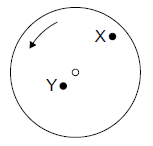Point X is a distance 2L from the centre of the disc. Point Y is a distance L from the centre of the disc. Point Y has a linear speed v and a centripetal acceleration a.

What is the linear speed and centripetal acceleration of point X?31. The mass of Earth is

ME, its radius is RE and the magnitude of the gravitational field strength at the surface of Earth is g. The universal gravitational constant is G. The ratio g/G is equal to

32. A communications satellite is moving at a constant speed in a circular orbit around Earth. At any given instant in time, the resultant force on the satellite is
33. The mass of a planet is twice that of Earth. Its radius is half that of the radius of Earth. The gravitational field strength at the surface of Earth is g. The gravitational field strength at the surface of the planet is
34. An aircraft is flying at constant speed in a horizontal circle. Which of the following diagrams best illustrates the forces acting on the aircraft in the vertical plane?
35. A pendulum bob is attached to a light string and is swinging in a vertical plane.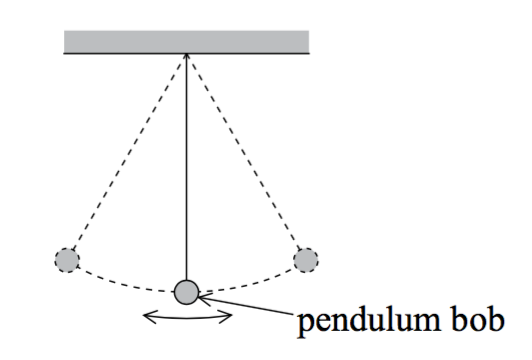At the lowest point of the motion, the magnitude of the tension in the string is

36. A car on a road follows a horizontal circular path at constant speed. Which of the following correctly identifies the origin and the direction of the net force on the car?37. Two pulses are travelling towards each other.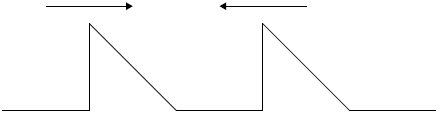What is a possible pulse shape when the pulses overlap?

38. Two satellites of mass m and 2m orbit a planet at the same orbit radius. If F is the force exerted on the satellite of mass m by the planet and a is the centripetal acceleration of this satellite, what is the force and acceleration of the satellite with mass 2m?39. A mass attached to a string rotates in a gravitational field with a constant period in a vertical plane.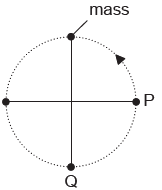How do the tension in the string and the kinetic energy of the mass compare at P and Q?40. A cyclist rides around a circular track at a uniform speed. Which of the following correctly gives the net horizontal force on the cyclist at any given instant of time?41. The force F between particles in gravitational and electric fields is related to the separation r of the particles by an equation of the form

F=(bc)/r^2

Which of the following identifies the units for the quantities a, b and c for a gravitational field?42. An electron moves with uniform circular motion in a region of magnetic field. Which diagram shows the acceleration a and velocity v of the electron at point P?
43. A mass connected to one end of a rigid rod rotates at constant speed in a vertical plane about the other end of the rod.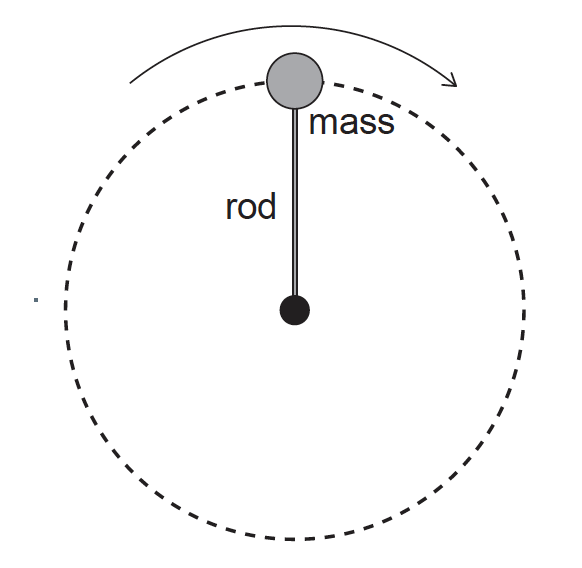The force exerted by the rod on the mass is

44. Two particles, X and Y, are attached to the surface of a horizontally mounted turntable.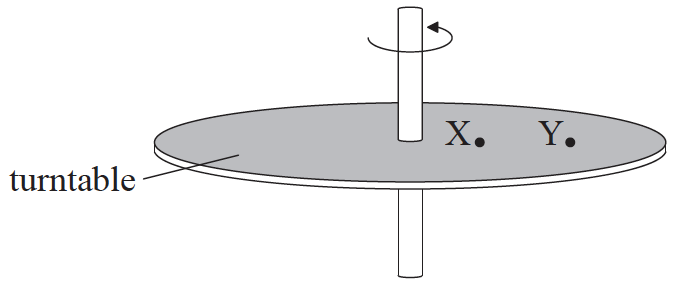The turntable rotates uniformly about a vertical axis. The magnitude of the linear velocity of X is v and the magnitude of its acceleration is a. Which of the following correctly compares the magnitude of the velocity of Y and the magnitude of the acceleration of Y with v and a respectively?